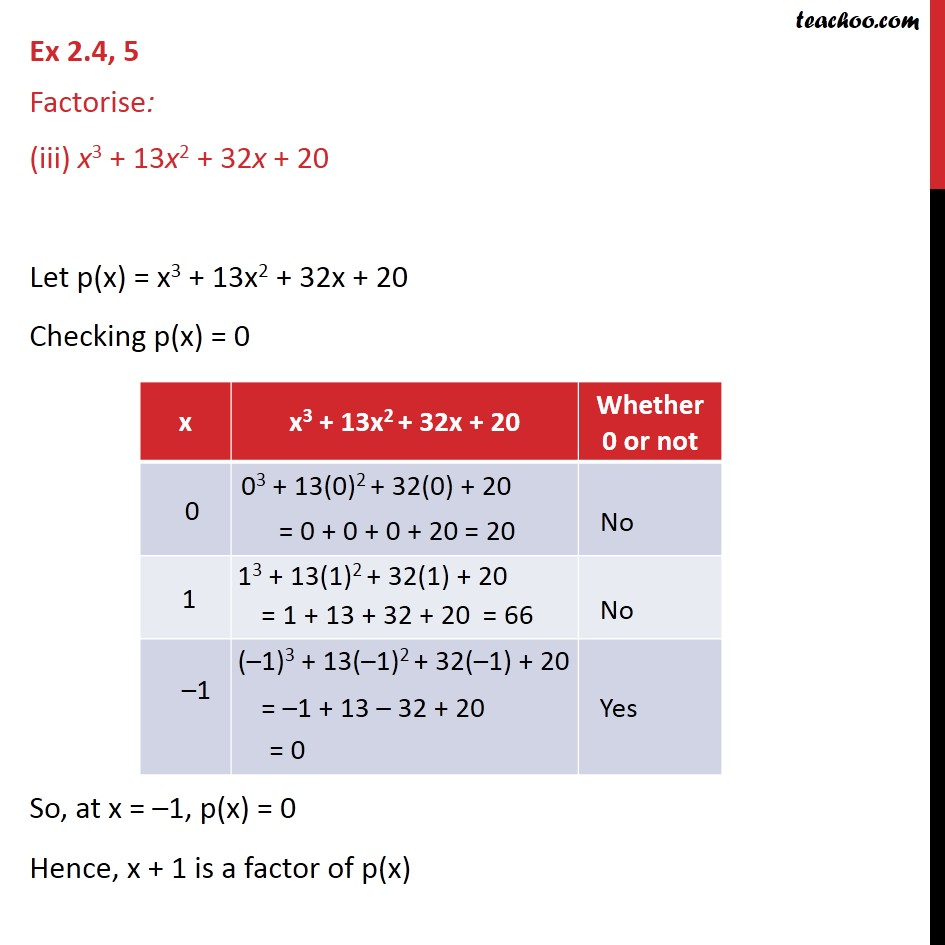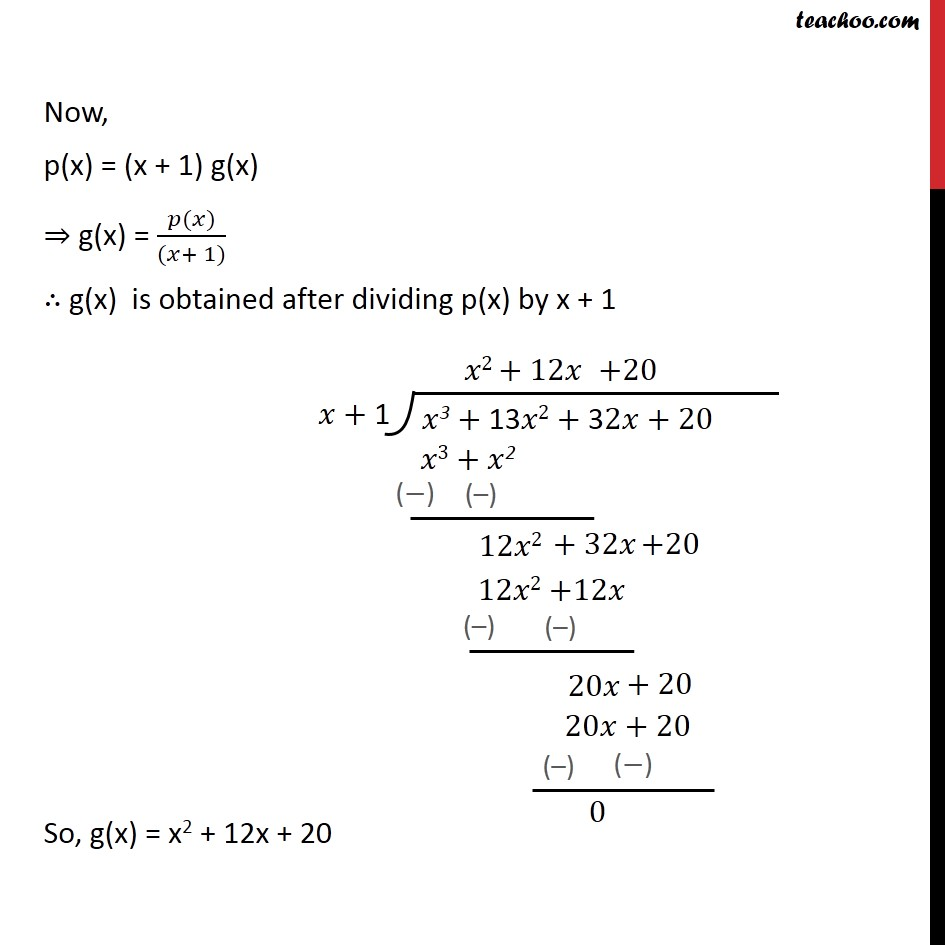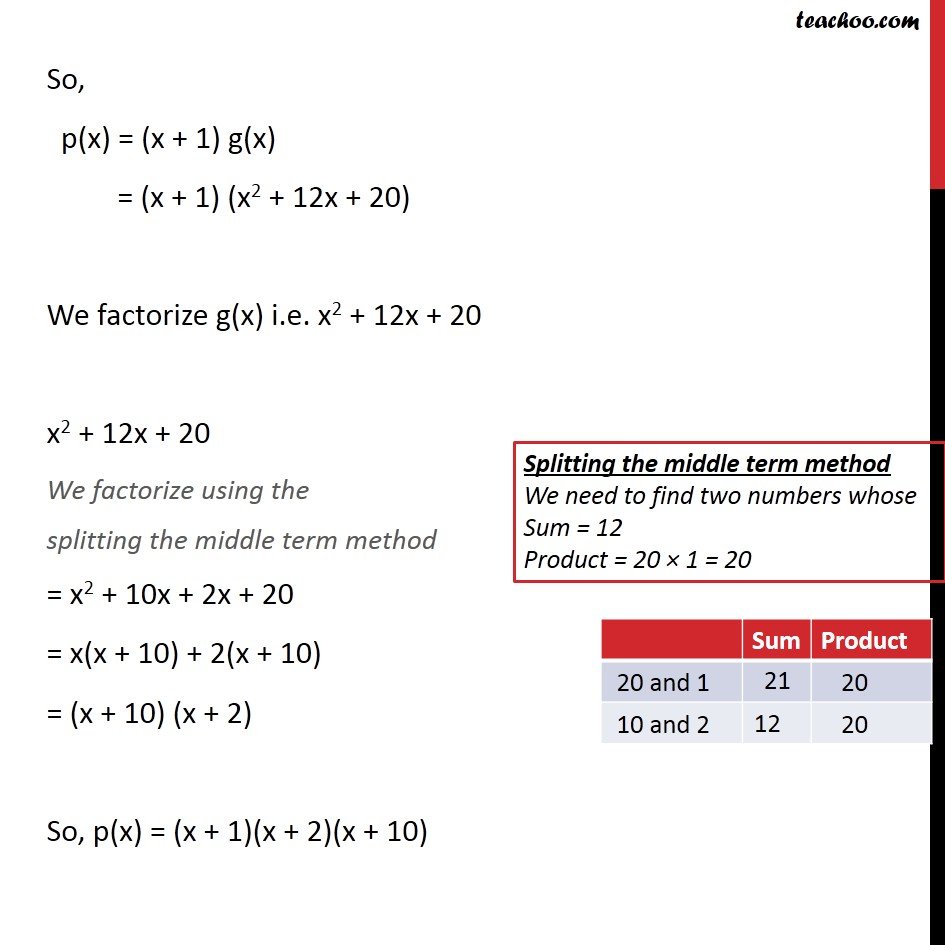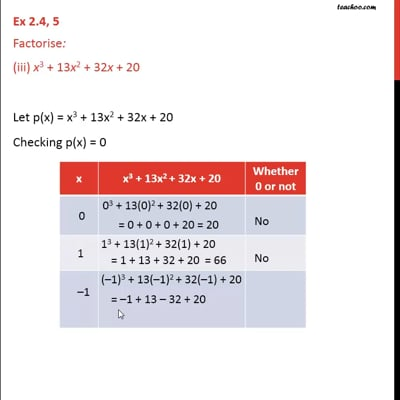Ex 2.4

Chapter 2 Class 9 Polynomials
Serial order wiseThis video is only available for Teachoo black users

Introducing your new favourite teacher - Teachoo Black, at only ₹83 per month

### Transcript

Ex 2.4, 5 Factorise: (iii) x3 + 13x2 + 32x + 20 Let p(x) = x3 + 13x2 + 32x + 20 Checking p(x) = 0 So, at x = –1, p(x) = 0 Hence, x + 1 is a factor of p(x) Now, p(x) = (x + 1) g(x) ⇒ g(x) = (𝑝(𝑥))/((𝑥+ 1)) ∴ g(x) is obtained after dividing p(x) by x + 1 So, g(x) = x2 + 12x + 20 So, p(x) = (x + 1) g(x) = (x + 1) (x2 + 12x + 20) We factorize g(x) i.e. x2 + 12x + 20 x2 + 12x + 20 We factorize using the splitting the middle term method = x2 + 10x + 2x + 20 = x(x + 10) + 2(x + 10) = (x + 10) (x + 2) So, p(x) = (x + 1)(x + 2)(x + 10)